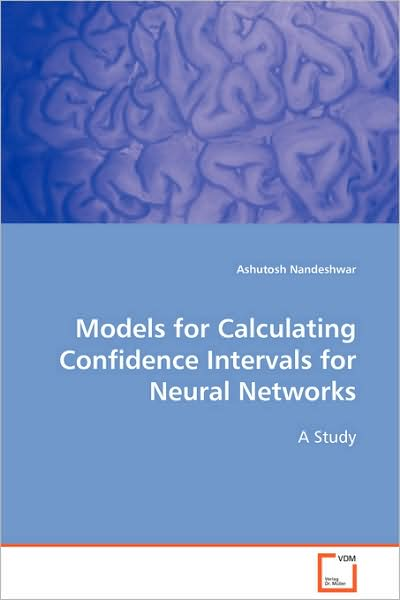## Ashutosh Nandeshwar

Pris
SEK 859
Förväntad leverans 23 jun - 3 jul

# Models for Calculating Confidence Intervals for Neural Networks

This books provides the methodology of analyzing existing models to calculate confidence intervals on the results of neural networks. The three techniques for determining confidence intervals determination were the non-linear regression, the bootstrapping estimation, and the maximum likelihood estimation. The neural network used the back­propagation algorithm with an input layer, one hidden layer and an output layer with one unit. The hidden layer had a logistic or binary sigmoidal activation function and the output layer had a linear activation function. These techniques were tested on various data sets with and without additional noise. The ranges and standard deviations of the coverage probabilities over 15 simulations for the three techniques were computed.

 Media Böcker     Pocketbok   (Bok med mjukt omslag och limmad rygg) Releasedatum 10 december 2008 ISBN13 9783639105483 Utgivare VDM Verlag Antal sidor 132 Mått 185 g Språk Engelska   Tyska